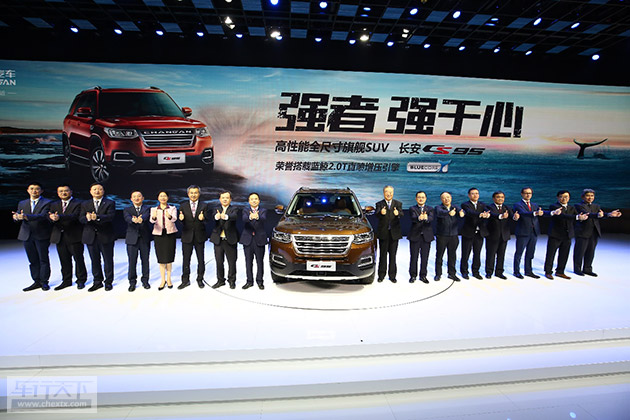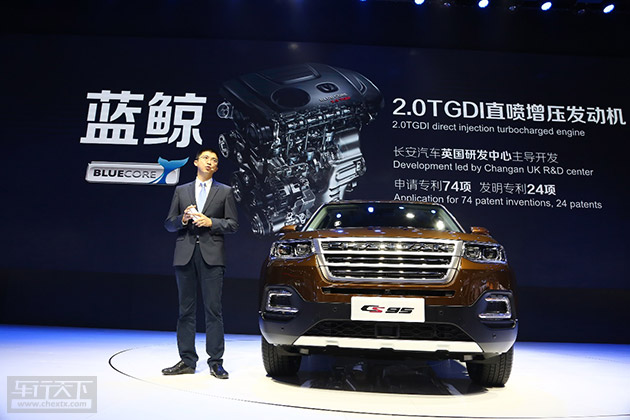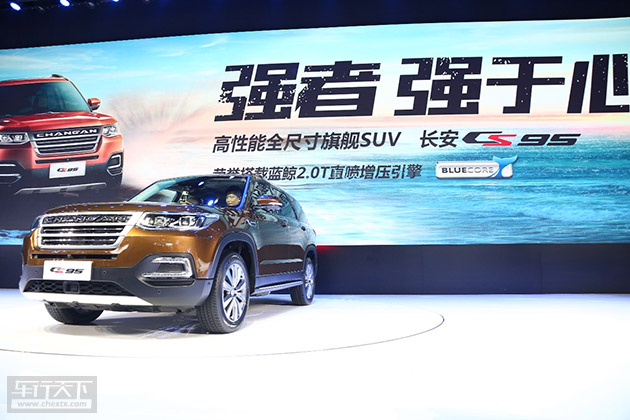## 强者强于心 长安CS95广州车展携最强发动机震撼来袭

2016年11月18日 10:50 来源：www.chextx.com 超过：次关注

11月18日，第十四届广州国际汽车展览会盛大开幕，长安汽车携旗下首款大型SUV长安CS95亮相3.1馆。此次长安CS95一经亮相就成为了车展舞台上的最耀眼的明星车型，各种长枪短炮频频聚焦在了CS95车尾的“360T”标识上，原因只有一个，那就是长安CS95搭载了长安汽车100%自主研发的中国最强蓝鲸2.0TGDI发动机。2.0TGDI

长久以来，中国汽车品牌对发动机一直是讳莫如深，发动机核心技术似乎成为了中国汽车品牌向上突破的天堑鸿沟，迟迟无法取得突破性进展。而此次搭载中国最强蓝鲸2.0TGDI发动机的长安CS95将彻底打破这一局面，从根本上直面主流合资品牌的挑战，引领中国汽车品牌回归汽车性能本质的全新时代。依托于长安汽车全球研发体系，集意大利艺术之都——都灵设计中心最时尚的设计语言、英国伯明翰动力研发中心和美国底特律底盘研发中心的顶尖全球智慧，协同博世、博格华纳、爱信等全球顶级的供应商伙伴，长安汽车倾力打造了CS95这款“高性能全尺寸旗舰SUV”。长安CS95的外观运用极简的设计语言，塑造内敛而富有张力的形态，彰显硬朗霸气的外在，同时也恰到好处的比例和精致的型面细节，匠心打造动感而优雅的灵魂；整车全LED灯具，目光如炬，与CS95的整体外观相契合，造就极致体验。长安CS95拥有4949*1930*1785mm的全尺寸车身，轴距达到2810mm，创造了无与伦比的真7座宽适SUV驾乘空间，尤其是第三排可变空间领先同级水平。另外还搭载了世界顶级的蓝鲸2.0TGDI直喷增压发动机，可实现最大233马力（171KW），在1750rpm-3500rpm之间持续爆发360N·m的最大扭矩，尤其是在1500rpm即可爆发最大扭矩的85%达到300N·m，急加速工况发动机在2000rpm左右可启发发动机超增压功能，瞬态可爆发最大380N·m的扭矩，配合强大的爱信6AT自动变速器液力增扭功能，起步瞬间可将发动机最大扭矩放大到720N·m，坡道起步及脱困时拥有超凡的动力,更为难得的是如此强大的动力仅需使用93号汽油即可实现。

这一最强“中国芯”的动力表现已达到国际品牌大型SUV发动机的同级水平。长安汽车历时6年时间，100%正向研发的蓝鲸2.0TGDI发动机，由人均研发经验超过25年的长安汽车英国研发中心主导开发，其精英研发团队大部分来自于捷豹、路虎、福特、宝马、劳斯莱斯、宾利等著名汽车企业。这款发动机共申请专利74项，其中发明专利24项，相关研究成果已通过国家863项目专家验收，填补国内2.0L及以上增压直喷发动机自主开发能力空白，是国内最强，世界领先的发动机。

同时， CS95的发动机台架和道路试验验证严格按照长安汽车试验验证体系CA-TVS进行开发，验证覆盖用户的各类常用工况和极限使用工况。4轮道路可靠性试验，40余台工装样车，总里程达到150万km以上，3轮次夏季、高原、冬季、高温、低温环境试验以及极寒极热舱环境适应性试验，发动机的可靠性和稳定性得到了进一步验证。

此外，这款集全球顶级智慧的蓝鲸2.0TGDI发动机在关键零部件上选择国际领先供应商产品,电喷系统全部零件供应商为德国博世,变排量机油泵为德国皮尔博格,活塞为美国辉门,发电机为法国法雷奥,正时轮系为美国盖茨, DVVT系统为德国INA,平衡轴为德国美特,机械真空泵为美国威伯科等,充分体现长安发动机开发团队协同国际供应商进行整机集成开发的能力。与这枚“中国最强芯”匹配的是全球领先的爱信TF-81SC 6速手自一体变速器。它具有高效的传递效率，具备丰富的控制功能，使车辆能更好地适应各种工况，能够在0.8秒之内实现快速换挡响应，尤其是复杂路况和越野路况，动感更足的手动模式还能够让喜爱挑战极限的越野爱好者纵享驾驶乐趣。

CS95的四驱车型采用国际领先的博格华纳全新一代NexTrac 智能四驱电子控制系统，具备强大的越野持续脱困能力。它可以根据道路工况合理分配前后轮扭矩，同时为车辆带来更高效的驱动性能和起步加速性。值得一提的是，长安汽车作为美国智能化联盟创始单位，无人驾驶智能技术达到了国际先进水平，而长安CS95将应用诸多最新的无人驾驶智能技术配置，如ACC自适应巡航系统、车道偏离预警系统，PAB预警辅助制动系统，智能并线辅助系统、自动泊车辅助系统等，让CS95的智能安全快人一步，高人一等，全方位智能呵护驾乘者的安全。一部车的功率要有多大，才足以驱动一个时代；一部车的扭矩要有多强，才足以扭转一种格局。一直以来长安汽车坚持自主研发与科技创新，致力于打造中国最强大且持续领先的研发体系。而这款中国最强蓝鲸2.0T发动机的研发成功，也将让长安汽车享受科技红利，巩固中国汽车品牌的领导者地位。凭借“蓝鲸2.0TGDI+ TF-81SC 6AT+ NexTrac”最优动力性能组合，拥有超强的极限脱困能力以及可靠性，发挥出动力系统“三剑客”的强大优势，长安CS95将引领中国汽车品牌回归汽车性能本质的新时代，擎动中国汽车品牌的第三次跃升。

#### 相关文章

0-500 字已有评论 0条 查看评论>>

﻿
• 快速找车
• 选择品牌
• 选择品牌
• A  奥迪
• A  阿斯顿·马丁
• A  阿尔法·罗密欧
• B  宝沃
• B  布加迪
• B  巴博斯
• B  保时捷
• B  宾利
• B  奔驰
• B  宝马
• B  本田
• B  别克
• B  标致
• B  比亚迪
• B  宝骏
• B  北汽制造
• B  北汽新能源
• B  北汽幻速
• B  北汽威旺
• B  北京汽车
• B  奔腾
• B  北汽绅宝
• B  北汽昌河
• C  长安欧尚
• C  长安
• C  长安凯程
• C  长城
• D  大众
• D  道奇
• D  DS
• D  东南
• D  东风风神
• D  东风风行
• D  东风小康
• D  东风风度
• D  东风
• F  福特
• F  丰田
• F  菲亚特
• F  法拉利
• F  福田
• F  福迪
• F  福汽启腾
• G  观致
• G  广汽传祺
• G  广汽吉奥
• G  GMC
• H  红旗
• H  汉腾汽车
• H  哈弗
• H  哈飞
• H  海格
• H  海马
• H  华颂
• H  黄海
• H  华泰
• H  恒天
• J  几何汽车
• J  捷达
• J  吉利汽车
• J  捷豹
• J  Jeep
• J  江淮
• J  江铃
• J  金杯
• J  九龙
• J  金旅
• K  凯翼
• K  凯迪拉克
• K  克莱斯勒
• K  科尼塞克
• K  卡威
• K  开瑞
• L  路虎
• L  林肯
• L  劳斯莱斯
• L  兰博基尼
• L  雷克萨斯
• L  铃木
• L  领克
• L  雷诺
• L  理念
• L  力帆
• L  莲花汽车
• L  猎豹
• L  路特斯
• L  陆风
• M  马自达
• M  MG
• M  MINI
• M  玛莎拉蒂
• M  摩根
• M  迈凯轮
• N  纳智捷
• O  欧拉
• O  欧宝
• O  讴歌
• O  欧朗
• Q  奇瑞
• Q  起亚
• Q  启辰
• R  日产
• R  荣威
• R  瑞麒
• S  SERES赛力斯
• S  三菱
• S  斯威汽车
• S  萨博
• S  smart
• S  斯柯达
• S  斯巴鲁
• S  思铭
• S  双龙
• S  上汽大通
• S  双环
• T  特斯拉
• T  腾势
• W  蔚来
• W  沃尔沃
• W  WEY
• W  五菱汽车
• W  五十铃
• W  威兹曼
• W  威麟
• X  现代
• X  雪佛兰
• X  星途
• X  雪铁龙
• X  小鹏汽车
• X  西雅特
• Y  一汽
• Y  英菲尼迪
• Y  英致
• Y  依维柯
• Y  野马汽车
• Y  永源
• Z  众泰
• Z  中华
• Z  中兴
• Z  知豆
• 选择车系
• 选择车系
• 车型对比
• 选择品牌
• 选择品牌
• A  奥迪
• A  阿斯顿·马丁
• A  阿尔法·罗密欧
• B  宝沃
• B  布加迪
• B  巴博斯
• B  保时捷
• B  宾利
• B  奔驰
• B  宝马
• B  本田
• B  别克
• B  标致
• B  比亚迪
• B  宝骏
• B  北汽制造
• B  北汽新能源
• B  北汽幻速
• B  北汽威旺
• B  北京汽车
• B  奔腾
• B  北汽绅宝
• B  北汽昌河
• C  长安欧尚
• C  长安
• C  长安凯程
• C  长城
• D  大众
• D  道奇
• D  DS
• D  东南
• D  东风风神
• D  东风风行
• D  东风小康
• D  东风风度
• D  东风
• F  福特
• F  丰田
• F  菲亚特
• F  法拉利
• F  福田
• F  福迪
• F  福汽启腾
• G  观致
• G  广汽传祺
• G  广汽吉奥
• G  GMC
• H  红旗
• H  汉腾汽车
• H  哈弗
• H  哈飞
• H  海格
• H  海马
• H  华颂
• H  黄海
• H  华泰
• H  恒天
• J  几何汽车
• J  捷达
• J  吉利汽车
• J  捷豹
• J  Jeep
• J  江淮
• J  江铃
• J  金杯
• J  九龙
• J  金旅
• K  凯翼
• K  凯迪拉克
• K  克莱斯勒
• K  科尼塞克
• K  卡威
• K  开瑞
• L  路虎
• L  林肯
• L  劳斯莱斯
• L  兰博基尼
• L  雷克萨斯
• L  铃木
• L  领克
• L  雷诺
• L  理念
• L  力帆
• L  莲花汽车
• L  猎豹
• L  路特斯
• L  陆风
• M  马自达
• M  MG
• M  MINI
• M  玛莎拉蒂
• M  摩根
• M  迈凯轮
• N  纳智捷
• O  欧拉
• O  欧宝
• O  讴歌
• O  欧朗
• Q  奇瑞
• Q  起亚
• Q  启辰
• R  日产
• R  荣威
• R  瑞麒
• S  SERES赛力斯
• S  三菱
• S  斯威汽车
• S  萨博
• S  smart
• S  斯柯达
• S  斯巴鲁
• S  思铭
• S  双龙
• S  上汽大通
• S  双环
• T  特斯拉
• T  腾势
• W  蔚来
• W  沃尔沃
• W  WEY
• W  五菱汽车
• W  五十铃
• W  威兹曼
• W  威麟
• X  现代
• X  雪佛兰
• X  星途
• X  雪铁龙
• X  小鹏汽车
• X  西雅特
• Y  一汽
• Y  英菲尼迪
• Y  英致
• Y  依维柯
• Y  野马汽车
• Y  永源
• Z  众泰
• Z  中华
• Z  中兴
• Z  知豆
• 选择车系
• 选择车系
• 选择车型
• 选择车型
• 意见反馈